: A chocolate problem that : Combinatorial Games and Beautiful : Introduction of the theory

# Another chocolate problem that is a variant of the game of Nim

Next we are going to study another chocolate problem that is a variant of the game of Nim, and this variant has a complicated mathematical structure.

Example 2.1.   This time we are gong to use the chocolate in Graph 2.1. This problem has been introduced by the authors.

Graph 2.1.The problem of Graph 1.2 is different from the problem of Graph 2.1. In Graph 2.1 you can cut the chocolate in 6 ways, so it is appropriate to represent it with 6 non-negative integers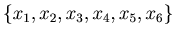. We represent the position in Graph 2.1 with {2,1,2,1,2,1}.

Note that these 6 coordinates are not independent, i.e., in some cases you cannot subtract a natural number from one of 6 coordinates without affecting other coordinates.
It is clear that we have 6 inequalities between these 6 coordinates.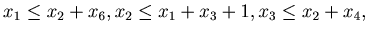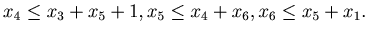We can study chocolates of any size if we use arbitrary non-negative integers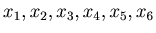that satisfy these 6 inequalities.

Example 2.2.   Here we are going to calculate P-positions of this game. Since there is no method to find all the P-position theoretically, we are going to find them by calculation of Mathematica. Because this game has a complicated structure, the Mathematica program for this game is a little bit complicated.
Clear[ss, al, allcases];
ss = 1; al =
Flatten[Table[{a,b,c,d,e,f},{a,0,ss + 2},{b,0,ss},{c,0,
ss + 2},{d,0,ss},{e,0,ss + 2},{f,0,ss}],5];
allcases =
Select[al,#[]+#[]+1>= #[]&&#[]+#[]>=#[[
3]] && #[]+#[]+1>=#[]&&#[]+#[]>=#[[
5]]&&#[]+#[]+1>=#[]&&#[]+#[]>=#[[
1]]&];(*allcases are the set of all possible shapes of the
chocolate.  Note that the above inequations are the necessary
and sufficient condtions for {a,b,c,d,e,f} to be a possible
shape of the cholocate.*)
num=Length[allcases];
(*num is the number of all the cases*)
x1=allcases[[num]];
(*x1 is the case with which we start the fame*)
pos[x_List,y_List]:=
Block[{s,t,u,v},u=x;v=y;t=Apply[Plus,v];
s=Position[v,t][[1,1]];
u[[s]]=u[[s]]-t;{Min[u[]+u[],u[]],
Min[u[]+u[]+1,u[]],
Min[u[]+u[],u[]],
Min[u[]+u[]+1,u[]],
Min[u[]+u[],u[]],
Min[u[]+u[]+1,u[]]}];
(*pos[x,y]returns the case that you will get after removing
y from x.
For example let x={1,2,1,2,1,2} and y={1,0,0,0,0,0},
then pos[x,y] denotes the case you get after removing one
from the 1st coodinate of x; that is {1,1,2,1,2,1}*)
Clear[move,z,p,t1,t2,t3,t4,t5,t6];
move[z_]:=Block[{p},p=z;
Union[Table[pos[p,{t1,0,0,0,0,0}],{t1,1,p[]}],
Table[pos[p,{0,t2,0,0,0,0}],{t2,1,p[]}],
Table[pos[p,{0,0,t3,0,0,0}],{t3,1,p[]}],
Table[pos[p,{0,0,0,t4,0,0}],{t4,1,p[]}],
Table[pos[p,{0,0,0,0,t5,0}],{t5,1,p[]}],
Table[pos[p,{0,0,0,0,0,t6}],{t6,1,p[]}]
]
]
(*move[x] returns all the cases you can get from x when
you remove a part of x*)
Mex[L_]:=Min[Complement[Range[0,Length[L]],L]];
Gr[z_]:=Gr[z]=Mex[Map[Gr,move[z]]];


By the Mathematica program in Example 2.2 we can find all the P-positions of the chocolate problem of Example 2.1 . (For this scale of problem you can find all the P-position only with pen and paper.) Graph 2.2 contains all the P-positions. All the other positions are N-position. As you can see easily the original position in Graph 2.1 is an N-position, because you cannot find the original position in Graph 2.2. Therefore you are sure to win if you start the game as the first player.

Graph 2.2.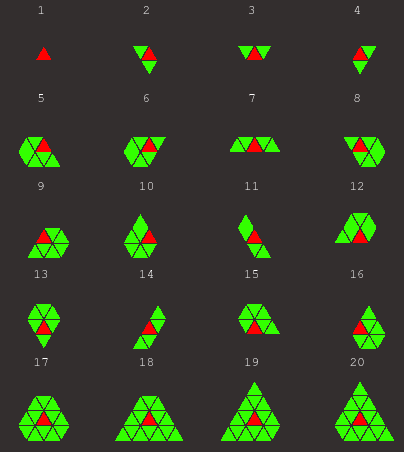Example 2.3.   Here we are going to study how to win the game as the next player. From the original position you can move to the 18th, 19th or 20th positions in Graph 2.2. Note that all of them are P-positions. Suppose that you chose the 18th position. Then your opponent will choose one of the 8 positions in Graph 2.3. Since they do not belong to the list of positions in Graph 2.2, all of positions of Graph 2.3 are N-positions.

Graph 2.3.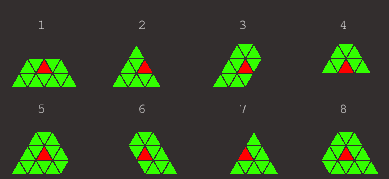If the opponent chose the first position of Graph 2.3, then you can choose the 7th of Graph 2.2. Then your opponent choose one of the 4 positions in Graph 2.4. If the opponent choose the 1th or the 3rd position, you can take away the green part and win the game. If the opponent choose the 2nd or 4th position of Graph 2.4, you can move to the 3rd of Graph 2.2. After that the opponent will take the right or left green part, then you can take the remainning green part and win.

The stratedy is clear. If you start with the original position in Graph 2.1, then you have only to move to a P-position. By the next move your opponent will move to an N-position. From this position you have only to move to a P-position. By continuing this process you are sure to win.

Graph 2.4.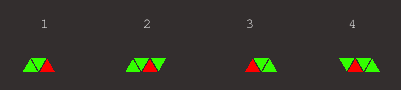Remark 2.1.   If you are going to play this game and want to memorize all the P-positions, it is better to make the list of P-positions smaller. In Graph 2.2 the 2nd, 3rd and 4th positions are essentially the same, and the 6th and 8th positions are essentially the same, too. By considering this we can choose 7 positions out of 20 positions in Graph 2.2, and we can make Graph 2.5.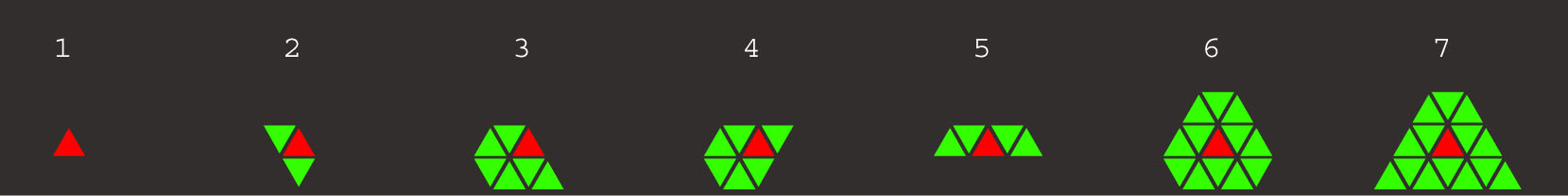We are going to study a bigger chocolate problem.

Example 2.4.   Suppose that you have the following chocolate. See Graph 2.6. The rule is the same as in the previous problem.

Graph 2.6.By using computers we can find all the P-positions. We have 118 P-positions. Instead of displaying all the P-positions we are going to choose a part of them. We did the same thing when we made Graph 2.5. This time we choose 30 positions out of all P-positions. The chosen P-positions are presented in Graph 2.7. As you can see easily, the original position in Graph 2.6 is an N-position, because you cannot find the original position in Graph 2.7. Therefore you are sure to win if you start the game as the first player. The strategy is easy and you can use the same kind of stratedy you used in the previous problem.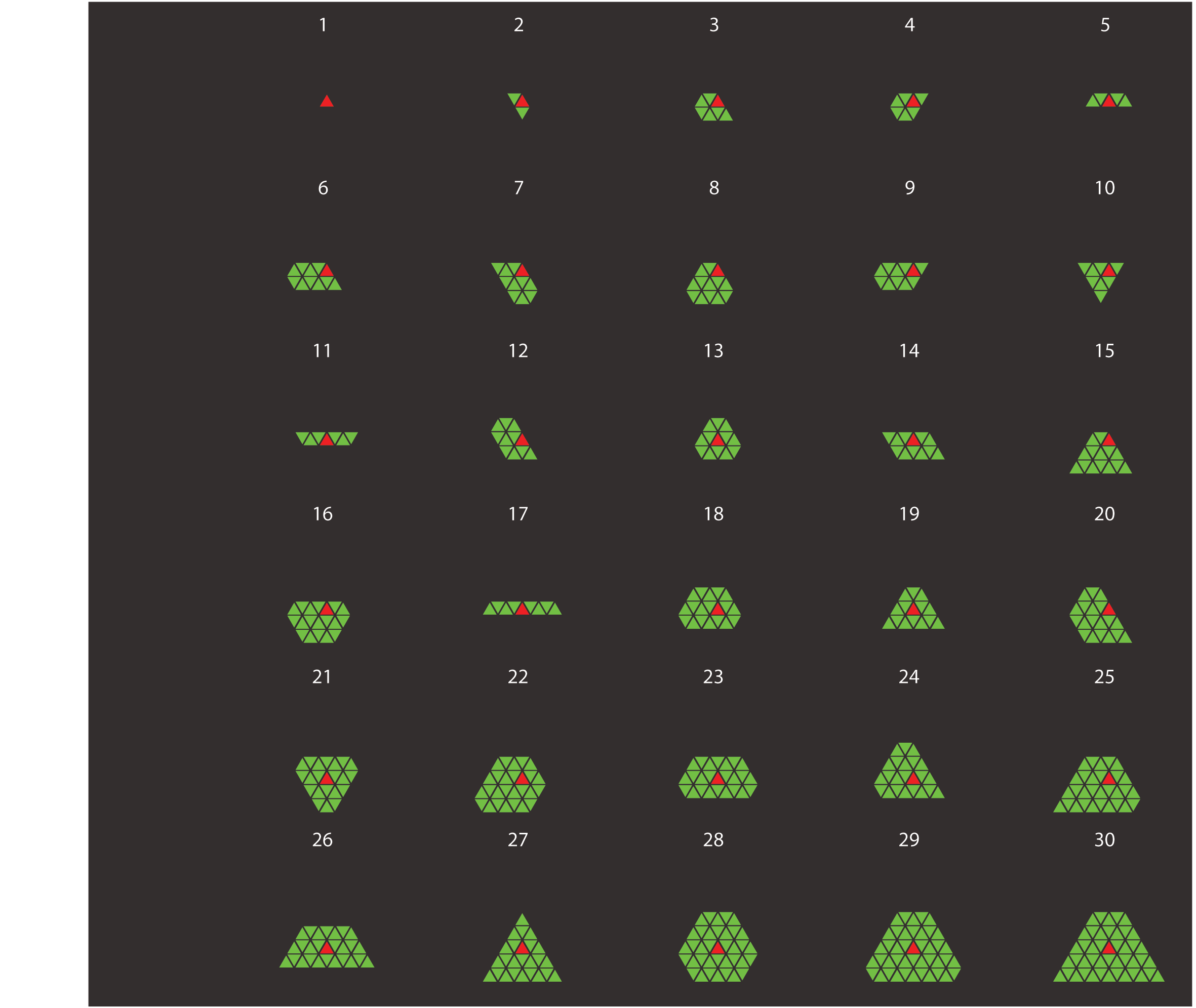: A chocolate problem that : Combinatorial Games and Beautiful : Introduction of the theory